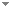Switch to: References

The Jordan curve theorem and the Schönflies theorem in weak second-order arithmetic

Archive for Mathematical Logic 46 (5-6):465-480 (2007)

 Erna and Friedman's Reverse Mathematics.Sam Sanders - 2011 - Journal of Symbolic Logic 76 (2):637 - 664.Elementary Recursive Nonstandard Analysis, in short ERNA, is a constructive system of nonstandard analysis with a PRA consistency proof, proposed around 1995 by Patrick Suppes and Richard Sommer. Recently, the author showed the consistency of ERNA with several transfer principles and proved results of nonstandard analysis in the resulting theories (see  and ). Here, we show that Weak König's lemma (WKL) and many of its equivalent formulations over RCA₀ from Reverse Mathematics (see  and ) can be 'pushed down' (...) Direct download (8 more)     Export citationBookmark5 citations Tanaka’s theorem revisited.Saeideh Bahrami - 2020 - Archive for Mathematical Logic 59 (7-8):865-877.Tanaka proved a powerful generalization of Friedman’s self-embedding theorem that states that given a countable nonstandard model \\) of the subsystem \ of second order arithmetic, and any element m of \, there is a self-embedding j of \\) onto a proper initial segment of itself such that j fixes every predecessor of m. Here we extend Tanaka’s work by establishing the following results for a countable nonstandard model \\ \)of \ and a proper cut \ of \:Theorem A. The (...)No categories Direct download (2 more)   Translate     Export citationBookmarkComplex Analysis in Subsystems of Second Order Arithmetic.Keita Yokoyama - 2007 - Archive for Mathematical Logic 46 (1):15-35.This research is motivated by the program of Reverse Mathematics. We investigate basic part of complex analysis within some weak subsystems of second order arithmetic, in order to determine what kind of set existence axioms are needed to prove theorems of basic analysis. We are especially concerned with Cauchy’s integral theorem. We show that a weak version of Cauchy’s integral theorem is proved in RCAo. Using this, we can prove that holomorphic functions are analytic in RCAo. On the other hand, (...) Direct download (3 more)     Export citationBookmark2 citations Formalizing Non-Standard Arguments in Second-Order Arithmetic.Keita Yokoyama - 2010 - Journal of Symbolic Logic 75 (4):1199-1210.In this paper, we introduce the systems ns-ACA₀ and ns-WKL₀ of non-standard second-order arithmetic in which we can formalize non-standard arguments in ACA₀ and WKL₀, respectively. Then, we give direct transformations from non-standard proofs in ns-ACA₀ or ns-WKL₀ into proofs in ACA₀ or WKL₀. Direct download (6 more)     Export citationBookmark5 citations Nonstandard Second-Order Arithmetic and Riemannʼs Mapping Theorem.Yoshihiro Horihata & Keita Yokoyama - 2014 - Annals of Pure and Applied Logic 165 (2):520-551.In this paper, we introduce systems of nonstandard second-order arithmetic which are conservative extensions of systems of second-order arithmetic. Within these systems, we do reverse mathematics for nonstandard analysis, and we can import techniques of nonstandard analysis into analysis in weak systems of second-order arithmetic. Then, we apply nonstandard techniques to a version of Riemannʼs mapping theorem, and show several different versions of Riemannʼs mapping theorem. Direct download (3 more)     Export citationBookmark2 citations